# Fraction Calculator

Fraction Calc is a special calculator for multiplication, division, addition, and subtraction of two or more fractions and whole numbers. It can process multiple fractions and whole numbers at once. Then it displays the step by step solutions of whatever operation it has processed. Sometimes few people will call it fraction solver, while others may say it is a mixed number calculator or mixed fraction calculator. It is an online calculator with fraction button. As of now it can compute up to ten both fractions and mixed numbers. It is useful for all students in all grade levels. It can be used as a reference to all math teachers and even those professionals who often use fractions in their workplace or in their homes.

a h
Simplify
Backspace
A/C
1
2
3
4
5
6
7
8
9
0
Whole Numbers
1
2
3
4
5
6
7
8
9
0
Numerators
1
2
3
4
5
6
7
8
9
0
Denominators
÷
x
+
=

## How To Use?

This calculator has been designed for easy use.

1. Press any number from the numerator buttons.
2. Press any number from the denominator buttons.
3. Press the add (+) button.
4. Press any number from the numerator buttons for the second fraction.
5. Press any number from the denominator buttons for the second fraction.
6. Press the equal (=) button to calculate the answer. Answer and solution will be displayed above.
• Adding three or more fractions
1. Repeat the steps above except the last step.
2. Press the add (+) button.
3. Press any number from the numerator buttons for the third fraction.
4. Press any number from the denominator buttons for the third fraction.
5. Press the equal (=) button to calculate the answer or press add (+) button to add more fractions.
6. The same process will be used to the fourth, fifth or any number of fractions. Just press the equal (=) button for the computation.
• Subtracting two, three or more fractions
• Multiplication and division of two, three or more fractions
• Follow the steps in adding fractions but instead of pressing add (+) button, press multiply (x) button for multiplication and divide (÷) button for division.
• Addition, Subtraction, Multiplication and Division of mixed numbers
• When dealing with mixed numbers, the important point to remember if you use this calculator is never forget to enter the whole numbers . The whole number buttons in the calculator is larger than the numerator and denominator buttons. You only need to press first the whole number button followed by fraction then you can proceed to any operation you want.

• Operations involving fractions, whole numbers, and mixed numbers
1. Press the whole number button if your fraction has a whole number or you can directly press the numerator button if you don’t need whole number. You cannot press denominator button if you have not pressed whole number or denominator button. This means that you need to press the whole number or numerator button first. Once numerator button is pressed, you can no longer press whole number button. You can only press whole number button again if you delete the numerator by pressing the backspace button. Zeroes should not be pressed first. Zeroes will be pressed after non-zero numbers are pressed.
2. Press denominator button for your denominator. Once pressed, you cannot press whole number or numerator button again. You can only press numerator button if you delete the denominator by pressing the backspace button.
3. Select any operation you want.
4. Press Equal button if you are done with your fraction. The solution will be displayed above.
5. Press Backspace if you want to delete one number at a time.
6. Press AC button to clear the fraction equation.
7. As of now, this calculator is limited only to 10 fractions.

## Fraction Calc on Android Mobile Phones

Our Fraction Calc for android mobile phones has been released. It can process basic and complex fraction operations and can display solution both in cross-multiply method and LCD (Least Common Denominator) method. You can get it from Google Play Store.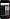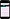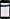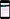## How Calculation Has Been Done?

Sometimes doubts about how the calculation is being made when multiple operations are being used. Using the MDAS notation, multiplication and division has the same precedence but higher than addition and subtraction. Addition and subtraction has the same precedence. Higher precedence is being processed first. This is always the rule and has been universally followed. While with same precedence, the operation is processed from left to right.

### Whole Number Fraction Calculator

Fraction Calc is also a whole number fraction calculator because it can deal a lot of whole numbers. Dealing with whole numbers means you have to study more and do extra steps by converting the whole numbers into a format suitable for mathematical operation. Doing mathematical operations with whole numbers means you have to do additional steps to get the correct answer. This means additional energy and burden for the people who were caught in the situation where they have to solve whole numbers and fractions. That is why several people are searching fraction and whole number calculator for the purpose of not only providing easy solutions for complex problems but also to save time and energy. Saving time and energy from certain task means that you gain extra resources to do an even more important task that would be very helpful.

### 3 Fraction Calculator

Most of the time only two fractions are involved in any mathematical arithmetic. It is very rare to have 3 fractions involved in any operation. But if ever this is your case, then you are very fortunate that you found this tool. You can easily use this tool as 3 fraction calculator because it can absolutely solve it. That is main purpose of this tool. Some people have not ever heard this tool so they have searched 3 fraction calculator specifically. But now that his tool has been created, I guess they may no longer have the time to worry at all.

### Multiple Fraction Calculator

Most of the calculators that have been created are limited in feature to the extent that it can only solve two fractions at a time. But Fraction Calc can even do more. It can solve up to 10 whole numbers or fractions combined. That is why many call it multiple fraction calculator. It is a very specialized calculator with whole numbers. The combination of whole number and fraction is hard to deal with but with this multiple fraction calculator the computation become easier. Adding mixed numbers, converting fraction to whole number, multiplying fractions by whole numbers, subtracting mixed numbers, and multiplying mixed fractions are among the processes this calculator can do.

1. It is easy to use.
2. It saves more time and energy.
3. There is no need for manual computation.
4. The computed result is accurate and precise.
1. It can make you dull in fraction calculation.
2. You will be very dependent on it in the future.
3. You may forget the rules of computation.

### Fraction Operation Rules

• Addition and Subtraction of fractions
• Addition and subtraction of fraction follows the same rules. They must have the same denominators to process the selected operation. You can add or subtract the two fractions if they have the same denominator, if not; you have to create a common denominator before adding or subtracting them.

Like fractions are fractions that have the same denominators. To add fractions with the same denominator add its numerator. For example, 2/5 + 1/5 = 3/5.

Fractions that have different denominators are unlike fractions. To add unlike fractions you need to make them have a common denominator. The simplest way to do it is using the butterfly method. To do the butterfly method, follow the steps below.

1. Multiply the numerator of the first fraction to the denominator of the second fraction. The result would be the first fraction numerator.
2. Multiply the denominator of the first fraction to the denominator of the second fraction. The result would be the new denominator of the first fraction.
3. Multiply the numerator of the second fraction with the denominator of the first fraction. The result would be the new numerator of the second fraction.
4. Multiply the denominator of the second fraction with the denominator of the first fraction. The result is now the new denominator of the second fraction.

For example: 2/3 + 3/5.

1. 2 x 5 = 10.
2. 3 x 5 = 15.
3. 3 x 3 = 9.
4. 5 x 3 = 15.

The new fraction are 10/15 and 9/15.
10/15 + 9/15 = 19/15.
The new fraction is 19/15.

To subtract fractions with the same denominator, just subtract the numerator of the second fraction from the numerator of the first fraction. Example: 4/6 – 3/6 = 1/6.

For fractions with different denominator, make them have a same denominator using the butterfly method and then do the subtraction after they have the same denominator.

• Multiplication and Division of fractions
• The rule of multiplying two fractions is straightforward. Multiply the numerator of first fraction to the numerator of the second fraction and multiply the denominator of the first fraction to the denominator of the second fraction. Example: 2/3 x 1/5 = 2/15.

To divide two fractions, you must first invert the second fraction then start multiplying the two fractions. Example: 2/3 divided by 1/5 = 2/3 x 5/1 = 10/3.

• How To Change Improper Fraction to Mixed Number
• When you reduce improper fraction to its lowest term, you need to change it to mixed number. This is done by dividing the numerator to denominator. The quotient will be the whole number. The remainder will be the new numerator and the denominator will remain as is.

• How To Change Mixed Number To Improper Fraction
• In dividing or multiplying mixed numbers, you need it to change into improper fraction. This is done by multiplying the whole number to denominator then adds the current numerator. The result will be the new numerator and the denominator will remain as is.

• Comparing Fractions
• For fractions that have the same denominators, the fraction that has the biggest numerator is the larger fraction than the fraction that has smaller numerator.
For fractions that have the same numerators, the fraction with the biggest denominator is smaller than the fraction that has smaller denominator.

• Simplifying Fractions
• From the topic above, we already know that there are equivalent fractions-fractions that are the same in value even if they have different numerators and denominators. Simplifying fraction means that using the smallest numerator and denominator but the same value. The fraction is in its simplest form when there is no common factor for numerator and denominator. For example, instead of using 7/14, we can use ½ which is the simplest form.

• Greatest Common Factor
• Greatest common factor is a largest number used to divide both numerator and denominator to get the simplest form of a fraction. For example, for fraction 12/30, the largest number to divide both the numerator and denominator is 6. By dividing it to 6, you will arrive to its simplest form which is 2/5.

Fractions are parts of a whole. For example there is one cake for five children. So the cake is divided into five parts. Each child would receive one part of the cake. The fraction would be 1/5. Each child will receive 1/5 of cake.There are two parts of fraction. The upper half part is called numerator. The lower half part is called denominator. Numerator is the part of the whole where it is being used or currently being dealt with.There are three types of fractions: proper fraction, improper fraction, and mixed numbers.

Proper fraction is a fraction where its numerator is always lesser than its denominator.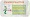Improper fraction is a fraction where its numerator is equal or greater than its denominator.Mixed Number represents whole numbers plus a fraction.Equivalent fractions are fractions that have different numerator and denominators but have the same value such as 1/2, 2/4, 7/14, 8/16, 10/20, 20/40, and 50/100.

## How Fraction Calc Was Created?

When I was a student, I have this math subject. One of the topics was about fraction. Although this topic is challenging, it greatly astonished me why it is hard to determine if the solution is right or wrong. You have to review it a number of times in order to be certain that your solution is right. It did not happen on me only. I found out that most of the students experienced the same. So, from there on, I dream that for one way or another, I will help them. I will help them to make sure that their solution is right without going through a lot of reviews. That is why I created this calculator. This calculator was created as a reference or a guide only to make sure that the student arrive the correct answer from their fraction problems. From The Founder of FractionCalc.com